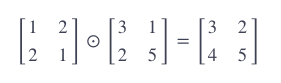# Tensorflow basics : Matrix operations

Matrix multiplication is probably is mostly used operation in machine learning, becase all images, sounds, etc are represented in matrixes.

There there are 2 types of multiplication:

## Element-wise multiplication : tf.multiply

Element-wise multiplication in TensorFlow is performed using two tensors with identical shapes. This is because the operation multiplies elements in corresponding positions in the two tensors. An example of an element-wise multiplication, denoted by the symbol, is shown below:``````import tensorflow as tf

A1 = tf.constant([1, 2, 3, 4])
B1 = tf.constant([3, 4, 5, 5])
C1 = tf.multiply(A1, B1)

# C1 = <tf.Tensor: id=2, shape=(4,), dtype=int32, numpy=array([ 3,  8, 15, 20], dtype=int32)>
``````

## Matrix multiplication : tf.matmul

``````import tensorflow as tf

A1 = tf.constant([[2, 24], [2, 26], [2, 57]])
B1 = tf.constant([, ])
C1 = tf.matmul(A1, B1)

# C1 = <tf.Tensor: id=5, shape=(3, 1), dtype=int32, numpy=
array([[ 5600],
[ 5900],
], dtype=int32)>
``````

Note, that matrixes should be exactly the same shape

``````import tensorflow as tf

A1 = tf.constant([1,2,3])
B1 = tf.constant([1,2,3])

# C1 = <tf.Tensor: id=20, shape=(3,), dtype=int32, numpy=array([2, 4, 6], dtype=int32)>
``````

## Matrix sum by dimension : tf.reduce_sum()

This operator just sum all elements of the matrix or specific row or column

``````import tensorflow as tf

A1 = tf.Variable([[1,2,3],[3,2,1], [3,3,3]])
B2 = tf.reduce_sum(A1)
# B2 <tf.Tensor: id=34, shape=(), dtype=int32, numpy=21>

B3 = tf.reduce_sum(A1, 0)
#B3 <tf.Tensor: id=37, shape=(3,), dtype=int32, numpy=array([7, 7, 7], dtype=int32)>

B4 = tf.reduce_sum(A1, 1)
#B4 <tf.Tensor: id=46, shape=(3,), dtype=int32, numpy=array([6, 6, 9], dtype=int32)>

``````